# ORF523: ISTA and FISTA

As we said in the previous lecture it seems stupid to consider that we are in a black-box situation when in fact we know entirely the function to be optimized. Consider for instance the LASSO objectivewhere one wants to minimize over. By resorting to black-box procedures one would solve this problem with Subgradient Descent and the rate of convergence would be of orderas this function is non-smooth (and potentially non-strongly convex). However we will see now that one can take advantage of the form of the LASSO objective which is a sum of a smooth part and a simple non-smooth part to obtain rates as fast as.

In this lecture (which follows this paper by Beck and Teboulle) we consider the unconstrained minimization of a sum of two functionsand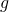that satisfy the following requirements:

(i)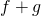admits a minimizeron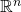.

(ii)andare convex, andis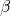-smooth.

(iii)is known andis accessible with a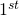-order oracle.

As we will see next, for the proposed algorithm to be computationally efficientalso needs to be ‘simple’. For instance a separable function (i.e.,) will be considered as a simple function. Our prime example will be.

ISTA (Iterative Shrinkage-Thresholding Algorithm)

Recall that the Gradient Descent algorithm to optimize the smooth functionis simply given bywhich can be written in the proximal form asNow here one wants to minimize, andis assumed to be known and ‘simple’. It seems very natural to consider the following iterative procedure known as ISTA:In terms of convergence rate it is not too hard to show that ISTA has the same convergence rate onthan Gradient Descent on, more precisely with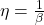one hasThis improved convergence rate over Subgradient Descent comes at a price: computing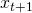may be a difficult optimization problem by itself in general, and this is why one needs to assume thatis ‘simple’. For instance ifcan be written asthen one can computeby solving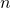convex problems in dimension. In the case where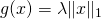this one-dimensional problem is given by: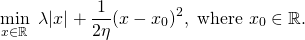Elementary computations show that this problem has an analytical solution given by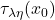, where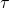is the shrinkage operator defined byFISTA (Fast ISTA)

As we have seen in this lecture, the optimal rate of convergence for smooth functions can be obtained with Nesterov’s Accelerated Gradient Descent. Combining this idea with ISTA one gets FISTA which is described as follows. LetLet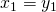an arbitrary initial point, andAgain it is not hard to show that the rate of FISTA is similar to the one of Nesterov’s Accelerated Gradient Descent, more precisely:This entry was posted in Optimization. Bookmark the permalink.

## 6 Responses to "ORF523: ISTA and FISTA"

••••